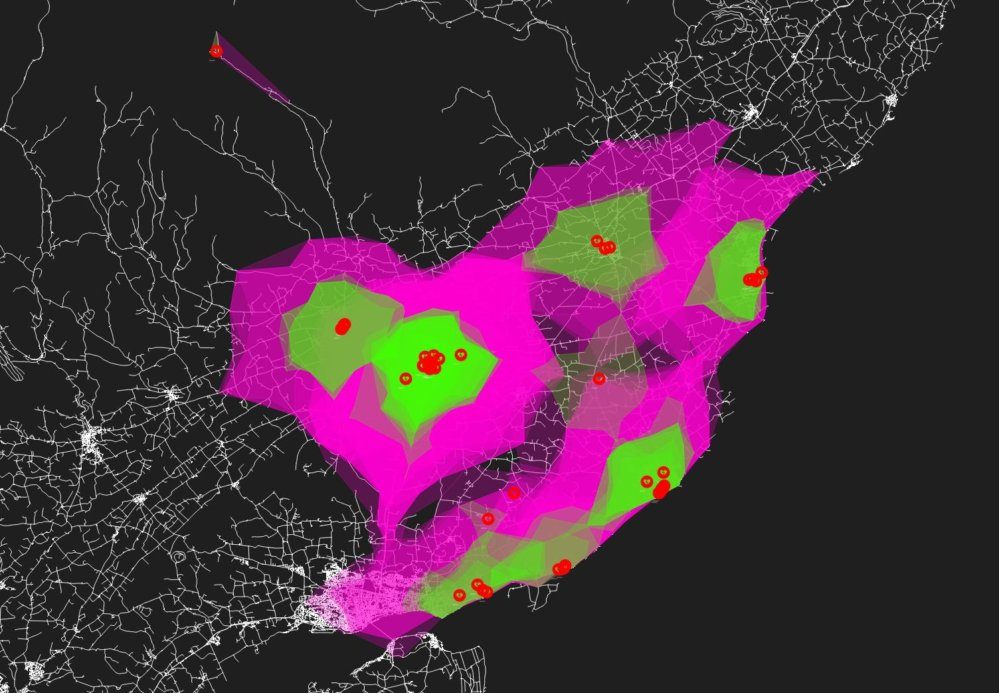# pgRouting alphashapes - batched

A while back I looked at generating alphashapes using pgRouting and displaying in QGIS. It was a pretty convoluted hack though and my SQL skills need some honing.

After seeing a post by smathermather that generated isochrones using PostgreSQL functions and the pgRouting algorithms I thought there must be a better way to generate my alphashapes. A use case of identifying access to defibrillator sets presented itself.

I looked at the code used by the pgRouting Layer QGIS plugin and stripped out the basic functionality. I used this to generate a temporary table of nodes representing the source and target nodes on the network.

``````CREATE TEMPORARY TABLE node AS
SELECT id,
ST_X(geometry) AS x,
ST_Y(geometry) AS y,
geometry
FROM (
SELECT source AS id,
ST_Startpoint(geometry) AS geometry
FROM itn_network --my network table
UNION
SELECT target AS id,
ST_Startpoint(geometry) AS geometry
FROM itn_network --my network table
) AS node;
``````

This table is then used in a function which can be passed any number of input points which it then uses to generate individual alphashapes for. The input points need some preparation as each point needs to be associated to the nearest node on the network. Taken from Anita Graser's work and adapted to my structure:

``````ALTER TABLE yourpoints

CREATE TEMPORARY TABLE temp AS
SELECT a.gid, b.id, min(a.dist)
FROM
(SELECT yourpoints.gid,
min(distance(yourpoints.the_geom, network_vertice_pgr.the_geom)) AS dist
FROM yourpoints, network_vertice_pgr
GROUP BY yourpoints.gid) AS a,
(SELECT yourpoints.gid, network_vertice_pgr.id,
distance(yourpoints.the_geom, network_vertice_pgr.the_geom) AS dist
FROM yourpoints, network_vertice_pgr) AS b
WHERE a.dist = b. dist
AND a.gid = b.gid
GROUP BY a.gid, b.id;

UPDATE yourpoints
SET nearest_node =
(SELECT id FROM temp WHERE temp.gid = yourpoints.gid);
``````

This assigns each input point a node id from the pgrouting vertices table. The function then uses the nearest_node value for each point as the starting point for the alphashape.

``````CREATE OR REPLACE FUNCTION public.make_isochrones()
RETURNS integer AS
\$BODY\$
DECLARE
cur_aed CURSOR FOR SELECT nearest_node FROM yourpoints WHERE nearest_node <> 35819;

v_nn cor_aed_sites.nearest_node%TYPE;
v_geom geometry;
v_sql varchar(120);

BEGIN
v_sql:='TRUNCATE TABLE public.yourisochrones';
EXECUTE v_sql; -- I truncate the table each time I run the function
OPEN cur_aed;
LOOP
FETCH cur_aed INTO v_nn;
SELECT ST_SetSRID(ST_MakePolygon(ST_AddPoint(foo.openline, ST_StartPoint(foo.openline))),27700) AS geometry --build polygon from line
FROM (
SELECT ST_Makeline(points ORDER BY id) AS openline --build line from points
FROM (
SELECT row_number() over() AS id, ST_MakePoint(x, y) AS points --get points from alphashape
FROM pgr_alphashape('
SELECT *
FROM node --get nodes making up alphashape
JOIN
(SELECT * FROM pgr_drivingDistance(''
SELECT gid AS id,
source::int4 AS source,
target::int4 AS target,
cost_time::float8 AS cost,
rcost_time::float8 AS reverse_cost
FROM itn_network'',
'||v_nn||', --loop through each input point
300, --adjust cost as necessary. Mine is in seconds.
true,
true)) AS dd ON node.id = dd.id1'::text)
) AS a
) AS foo INTO v_geom; --save the result geometry into variable

INSERT INTO public.isochrones(geometry) VALUES (v_geom); --insert into table

END LOOP;
RETURN 1;
CLOSE cur_aed;
END;
\$BODY\$
LANGUAGE plpgsql VOLATILE
COST 100;
``````

I created the table to hold the isochrones beforehand - a simple structure of id and geometry with a primary key and spatial index.

The results of a few hours work distilled down into 15 seconds runtime for 42 input points and a network of 72000 edges.Approximately 5 minutes travel time to the nearest Automated External Defibrillator [AED]Approximately 10 minutes travel time to the nearest Automated External Defibrillator [AED]

Provided you have someone at the scene of the cardiac arrest who is proficient in CPR and who can keep going for 20 minutes then if you're in the pink then you may be able to get the AED to save someone's life.

Note1: the list of AED devices shown here is not complete and not all are publicly accessible 24/7.